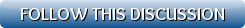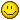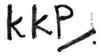Back to: Kurakani General Refresh page to view new replies[VIEWED 7701 TIMES] SAVE! for ease of future access.
helpjava11Posted on 12-11-14 9:38 AM     Reply [Subscribe]
Login in to Rate this Post:     0?Now I got all of yours attention, solve this problem.

A book has N pages, numbered the usual way, from 1 to N. The total number of digits in the page numbers is 1,095. How many pages does the book have?

instagramPosted on 12-11-14 10:17 AM     [Snapshot: 140]     Reply [Subscribe]
Login in to Rate this Post:     0?Jhan bihana bihana ke dekhna paaiyela bhanera kholeko ta. Daka le jhukkai halyo ni

sajhamitraPosted on 12-11-14 10:28 AM     [Snapshot: 153]     Reply [Subscribe]
Login in to Rate this Post:     0?N(N+1)/2=1095.. Solve for N. that's the answer

SlackdemicPosted on 12-11-14 10:41 AM     [Snapshot: 204]     Reply [Subscribe]
Login in to Rate this Post:     0?From 1 to 9 ====> 9 digits
10 to 99 =====> 180 digits
.                           + ----------
Total of 1 digit and 2 digit no.s  = 189 digits
Now rest of the pages are the three numbered pages:
N - 189 = 1095 - 189 = 906
906/3 = 302

So the total pages: 9 + 90 + 302 = 401
Last edited: 11-Dec-14 11:34 AM

sara_solta11Posted on 12-11-14 11:19 AM     [Snapshot: 309]     Reply [Subscribe]
Login in to Rate this Post:     0?1 to N = 1 to 129

helpjava11Posted on 12-11-14 1:13 PM     [Snapshot: 523]     Reply [Subscribe]
Login in to Rate this Post:     0?Slackdemic got it right. Answer is 401.

Todays score:
Slackdemic +1
Sajhamitra -1
sara_solta11 -1

virusno1Posted on 12-11-14 1:35 PM     [Snapshot: 553]     Reply [Subscribe]
Login in to Rate this Post:     0?Here is my tricky question.
You have range of contiguous numbers in array from 1 to 1 million. Among of them one of the numbers is missing. Find out that missing number. You can use any data structure or use a simple math.

aaiteyPosted on 12-11-14 3:04 PM     [Snapshot: 659]     Reply [Subscribe]
Login in to Rate this Post:     0?अहिलेको जमानामा यसो हल्का गुगल गर्नु पर्छ क्या, गुला खेलाउदै दिमाग चलाउन खोजेर मात्र हुदैन।int getMissingNo (int a[], int n)
{
int i, total;
total  = (n+1)*(n+2)/2;
for ( i = 0; i< n; i++)
total -= a[i];
}

/*program to test above function */
int main()
{
int a[] = {1,2,4,5,6};
int miss = getMissingNo(a,5);
printf("%d", miss);
/*getchar();*/
}
Last edited: 11-Dec-14 03:05 PM

sajhamitraPosted on 12-11-14 3:40 PM     [Snapshot: 711]     Reply [Subscribe]
Login in to Rate this Post:     0?Do it with a datastructure.

helpjava11Posted on 12-12-14 10:22 AM     [Snapshot: 1337]     Reply [Subscribe]
Login in to Rate this Post:     0?if the numbers are in sequence, this function works fine. if its not in sequence its different story.

main method(){
int[] arrayofMillionNumbers = new int;
for(int i = 1 ; i< arrayofMillionNumbers+1 ; i++){
}
findMissing(arrayofMillionNumbers);
}
private int findMissing(int[] num) {
int missingNumber = 0;
for (int i = 0; i < (num.length - 1); i++) {
if ((num[i + 1] - num[i]) > 1) {
missingNumber = num[i] + 1;
break;
}
}
return missingNumber;
}

neurologistPosted on 12-12-14 10:31 AM     [Snapshot: 1348]     Reply [Subscribe]
Login in to Rate this Post:     0?if the numbers are in sequence, this function works fine. if its not in sequence its different story.

m = missing number
x = 1;
for i = 1 to million
{
if x is not equal to i
{
m = x = missing number;
stop iteration;}
x = x + 1;
}
output m;

sajhamitraPosted on 12-12-14 10:42 AM     [Snapshot: 1369]     Reply [Subscribe]
Login in to Rate this Post:     0?those numbers are continuous but distributed randomly. like 1,3,2,5,4.....etc
your solution works only if numbers are continuous but there should be some efficient way to solve if sequential numbers are randomly distributed.
Last edited: 12-Dec-14 10:44 AM

KaliKoPoiPosted on 12-12-14 10:46 AM     [Snapshot: 1378]     Reply [Subscribe]
Login in to Rate this Post:     0?Mr. DPosted on 12-12-14 11:38 AM     [Snapshot: 1412]     Reply [Subscribe]
Login in to Rate this Post:     0?Add all the numbers in the array.
Then find n! ( that is 1,000,000!)
Subtract them, that would be number that is missing.

This is would be complete in O(n) which is better than sorting the number and then using one of above algorithm to find the missing one.

virusno1Posted on 12-12-14 12:43 PM     [Snapshot: 1460]     Reply [Subscribe]
Login in to Rate this Post:     0?Yes that's true but there is another solution too. Hint: You can use an array.